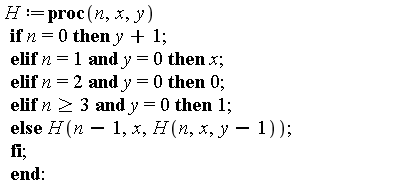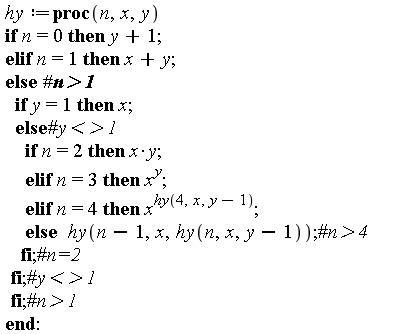## Yiannis Galidakis

Yiannis Galidakis

## 140 Reputation

7 years, 194 days
Agricultural University of Athens
Doctoral student
Athens, Greece

NULL

## How to make a recursion return a symboli...

Maple 13

Please refer to this for the definition(s):

http://www.mrob.com/pub/math/largenum-3.html#hyper

The hy(a,n,b) recursion turns  into the following Maple code:Unfortunately this code depends on x and y being explicit numeric values (x,y \in N), otherwise the recursion crashes (if I ask for example H(2,x,y) or H(2, x, 2))

Is there any way to transform the code so that the final construct can be shown symbolically?

I don't see anything obvious, especially since if y is not a specific natural number the recursion will crash. Can we maybe force Maple to not evaluate it for natural number arguments and return the final construct as either a sum, product or tower of the symbols for x and y (i.e. symbols of the digits of x and y like a tower of 2's 3's, etc)?

I do remember something about putting primes around functions prevents premature evaluation, but in this case it doesn't do anything like I'd want. This definition is not primitive recursive (like that of the Ackerman function), so I don't expect it to be able to be called abstractly (H(2,x,y) or something else such), but maybe we can turn it into something that shows the structure of the final construct as symbols of the digits of x and y?

Thanks.

Yannis

PS: One can improve the situation a bit, by implementing the extended definition as:

hy := proc (n, x, y) if n = 0 then y+1 elif n = 1 then x+y else if y = 1 then x else if n = 2 then x*y elif n = 3 then x^y elif n = 4 then x^hy(4, x, y-1) else hy(n-1, x, hy(n, x, y-1)) end if end if end if end proc(which can be called abstractly for n=0,1,2,3,4 (hy(n,x,m) for natural m), but for higher n it crashes similar to H, since the definition falls back to the previous if n>4.

## Can we improve on the accuracy of this f...

Maple 18

I am trying to reproduce this fractal graphic by Daniel Geisler, using maple 18:

http://tetration.org/Tetration/index.html

I suspect the images were produced by fractint - with a floating point hardware accelerator, but I am not sure. In any case, they were produced by a numerical program, not a symbolic one and probably with 16-18 digits of accuracy.

The Maple code I use makes for a valiant effort, but is nowhere close in accuracy to the two images above. Here's the Maple 18 code:

tetrationFractal18.mw

Several questions here:

1) Geisler's image colors according to period (right graph). Period 1 is red. 2 is yellow (although not all yellow regions are period 2) 3 is green, 4 is cyan, 5 is blue, etc.

I have some period checking code in my doc, which stores the last 20 iterates of the maxIter iterations of the orbit in orb[n]  then extracts a period p, based on some backwards comparisons.

I am only interested in graphing max period up to N=20, hence the orb[1..N] array, stores only the last N elements of the iterates. This is relatively fast, but extracting the period (below) from the orb array, puts an additional strain on the overall calculations, probably of the order of O((N+p)*n) for each n in the iteration, where p is the period returned. That's why I avoid storing in orb unless the global bound "bail" has not been exceeded.

The period finding algorithm works. It rerurns p correctly, and that's the value the graphic proc returns for plot3d. The problem is that the range of values is small (p\in[1..N]) and therefore the palette in plot3d is poor. I.e., it doesn't differentiate very much between colors of different period regions. How can I spread out the color pallete with plot3d with such results? ideally, I'd like to assign colors roughly from red to violet, with period 1 red, 2 orange, 3 green, 4 blue, 5 purple, etc.

I tried returning log(p) and this spreads the zhue some, but not enough (see attached document). Further, I'd like to assign black to points which escape the calculations, such as when the bail bound is exceeded in my iteration loop. By default, these show up as "purple", which is the same as the color for period 1 with the latest implementation. How can I except these escape points from the palette, i.e. what does the fractal proc have to return to color these black? Note that for these points, orb[n] defaults to 0, so the palette colors this close to p=1 region (these are the black "hairs"/threads of the Cantor Bouquets in Geisler's second image. That's the first question.

Second question is, why are there "white" regions interlaced under this scheme? is the calculation failing at these points because of low resolution? (epsilon~0.01 in this doc). (If points fail "bound"=1e10 then the orbit is orb[1..N]=0, so p=1 and zhue=1?) White seems to decrease as I increase epsilon and maxIter, but i can't test further, because the calculation limit is already prohibitive for this version of Maple. The graph with a plot3d grid [400,400] and maxIter=100 and epsilon=0.01, takes around 25 minutes. Testing anything finer than than, is time uneasonable.

Would increasing Digits help with any of the above?

Does anyone have any ideas on how to improve the code performance for this graph in maple 18, as per the above problems?.

Many thanks.

## w-plane Maple mapping does not agree wit...

Maple 18

I created some simple maple 9 code to do some z->w mappings long time ago, on my web page:

https://ingalidakis.com/math/ComplexMaps.html

Upon revising my webpage, I've stumbled upon a page for comformal mappings, by David Bau:

http://davidbau.com/conformal/#exp(z)

Here's the current version of my code in Maple 18:

rrestart;
NULL;
with(plots);
xMax := 1;
yMax := 1;
N := 10;
step := abs(xMax)/N;
GL := proc (x, y) options operator, arrow; x+I*y end proc;
f := sin;
G := {};
for k from -N+1 to N+1 do

G := union(G, {complexplot(f(GL(x, (k-1)*step)), x = -xMax .. xMax, color = brown)});

G := union(G, {complexplot(f(GL((k-1)*step, y)), y = -yMax .. yMax, color = brown)})

end do;
display(G, scaling = constrained);

The code is pretty simple: I am simply scanning the 1x1 unit square complex grid and create a complex plot of it on the w-plane, by passing it through whatever function is at: f:=x.

Does anyone have any idea why the grid lines on the w-plane do not agree with Bau's grid lines mapping of the w-plane? To try it for exp for example, input "exp(z)" in Bau's home page.

The difference is quite noticeable, especially on exp and log, where the orientation of the w-grid on the left and right half-planes, seem to be opposite of what my code displays.

The reason may be Mathematical, but from what I see from the description on Bau's page, there's no difference on the identity function, so I am clueless.

## Problem with involution...

Maple 18

Hi everyone,

Consider this maple 18 doc: Euler18.mw

The code is regular code for Julia sets of the exponential.

To see how the Julia set behaves at infinity, I apply the transform mu(z)=1/z.

The plot3d command correctly plots the Julia set at an appropriate neighborhood of infinity, but:

1) Axes are not transformed

2) Saving as .eps produces an empty plot, sans the axes (plot is saved correctly, when not applying mu(z))

Is there any trick to force the axes to also show correctly with the transformed ranges?

Seems that this misalignment is bothering the .eps renderer, which probably plots the graph in twilight zone, given the false ranges of the untransformed axes.

Any ideas on how to force the saveas .eps to work in this case?

Many thanks,

Yiannis

## Zooming-in with no axes, again......

Maple 18

Hi everyone,

Consider this Maple 18 doc:

FareySeq.mw

I am having the same problem as in:

http://www.mapleprimes.com/questions/203593-Maple-13-Fast-Maple-18-Crawling#comment213548

Namely, the produced animated .gif contains only one frame. Animation doesn't work, even though the button of the animation is active after I click on frame #1.

I have acer's solution at the end, but it doesn't work in this case either.

Breaks in both Maple 13 and 18.

TIA,

Yiannis

 1 2Page 1 of 2
﻿